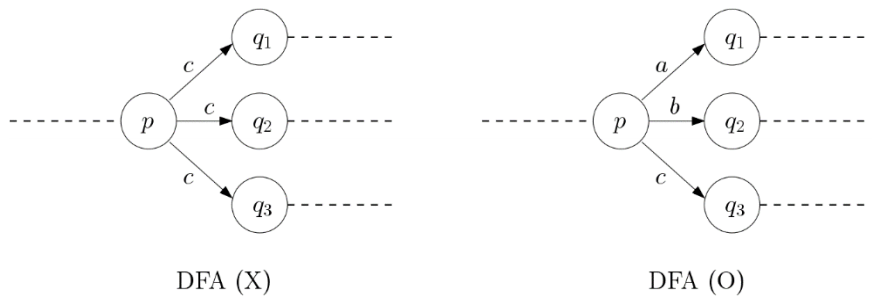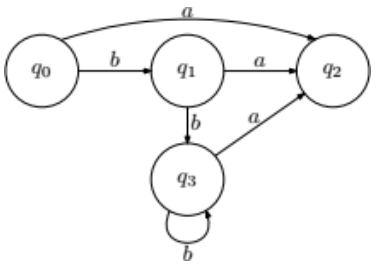시간 제한메모리 제한제출정답맞힌 사람정답 비율
2 초 512 MB0000.000%

## 문제

A deterministic finite automaton (DFA) is a labeled directed graph. That is, instead of having a directed edge in the form (p, q), edges are given as a triple (p, q, c), where p and q are nodes in the graph and c is a label character from an alphabet. At each node, there is at most one outgoing edge per character. In other words, there is no subset of edges in the form (p, q1, c), (p, q2, c), (p, q3, c), … of size greater than 1. On the other hand, a node can have a several outgoing edges all of which have different labels. In a DFA, one node is designated as the starting node q0 and a subset F of nodes are designated as final nodes.A DFA is specified by a tuple(N, K, M, q0, F), where N is a set of states, K is an input alphabet, M is a set of transitions, q0 is the start state and F is a set of accepting states. Given a DFA D and a string w = w1w2wn, we say that D accepts w if there is a series of edges (q0, q1, w1), (q1, q2, w2), … , (qn−1, qn, wn) spelling out w and qn is a final node in F. Note that nodes and edges can be visited multiple times. In this sense, a DFA can store a (possibly infinite) set of strings, namely the set of all strings accepted by the DFA. DFAs are useful in several practical applications. For instance, in software verification or pattern matching, often a target object is represented as a DFA for efficient processing.

Given two strings x and y, we say that y is a suffix of x if there is another string u such that x = uy (the concatenation of u and y in order). For example, for a string icpc2018, all strings icpc2018, cpc2018, pc2018, c2018, 2018, 018, 18, 8, λ are suffixes of icpc2018, where λ denotes the empty string. A set of strings is suffix-free if there is no pair of distinct strings x and y in the set such that y is a suffix of x. For instance, {2018, 18, icpc} is not suffix-free since 18 is a suffix of 2018. Similarly {λ, icpc2018, icpc} is not suffix-free since λ is a suffix of icpc2018 and icpc. In fact, λ is a suffix of all nonempty strings and, thus, any set containing λ and any other nonempty string is not suffix-free. Also, by definition, empty set is always suffix-free.

Given a DFA D, the language L(D) of D is the set of strings accepted by D. Then we say that D is suffix-free if there are no two distinct strings x and y in L(D) such that y is not a suffix of x. Suffix-freeness plays an important role in several applications including efficient pattern matching. If no string is accepted by D, then L(D) is empty and, therefore, is suffix-free.Your task is to determine whether or not a given DFA is suffix-free. In the right figure, the arrows correspond to labeled edges (transitions) in M. For example, there is an edge (q0, q2, a) and (q3, q3, b). Assume that q2 is the only final node in the DFA; namely, F = {q2}. The string a is accepted as there is a path (q0, q2, a). Furthermore, bbba is also accepted by (q0, q1, b), (q1, q3, b), (q3, q3, b), (q3, q2, a). Since a is a suffix of bbba, this DFA is not suffix-free. Note that these are not the only examples. For all inputs, you can assume that all nodes in the DFA are reachable from the starting node.

## 입력

Your program is to read from standard input. The input starts with a line containing four integers n, m, k, f, where n (1 ≤ n ≤ 2,000) is the number of nodes, k (1 ≤ k ≤ 26) is the number of characters in the alphabet, m (1 ≤ mkn) is the number of edges and f (1 ≤ f ≤ 2,000) is the number of final nodes. The nodes in the graph are labeled from 0 to n − 1, where 0 is the start node. The alphabet consists of the first k lowercase English letters. The following line contains f integers, separated by spaces, corresponding to the final node labels. The following m lines cointain two integers p and q and a character c, all separated by spaces, per line, which corresponds to the labeled edge (p, q, c).

## 출력

Your program is to write to standard output. Print exactly one line which contains 1 (if D is suffix-free) or 0 (otherwise).

## 예제 입력 1

4 6 2 1
2
0 2 a
0 1 b
1 2 a
1 3 b
3 2 a
3 3 b


## 예제 출력 1

0


## 예제 입력 2

4 7 3 1
3
0 1 a
0 2 b
0 3 c
1 1 b
1 3 a
2 2 c
2 3 b


## 예제 출력 2

1